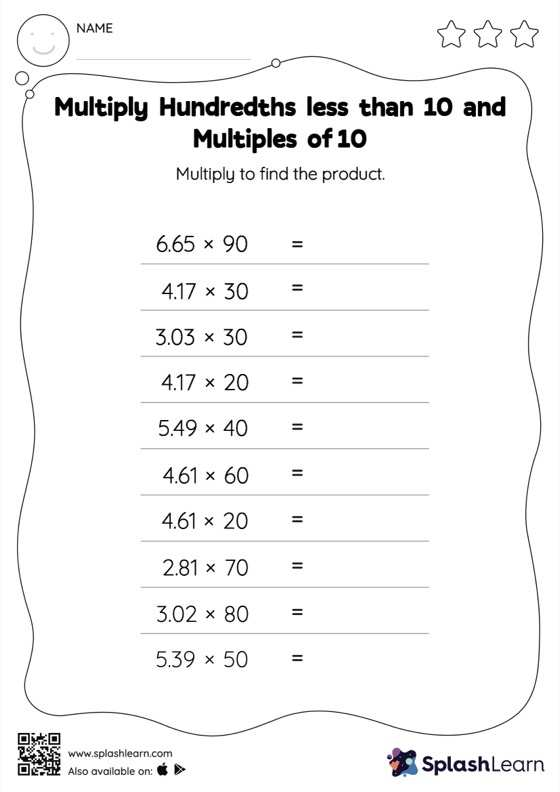# Multiply Hundredths less than 10 and Multiples of 10: Horizontal Multiplication Worksheet

Home > Multiply Hundredths less than 10 and Multiples of 10: Horizontal MultiplicationStudents know that when multiplying a decimal by a number ending in zeros, the decimal point is first ignored and then placed in the product as many places from the right as the number of decimal digits in the original number. They practice this idea thoroughly in multiply hundredths less than 10 and multiples of 10 worksheet. As the worksheet uses a horizontal format, it allows for more creativity when it comes to how the student solves the problem. In contrast, in the vertical format, students usually employ the standard method to solve.